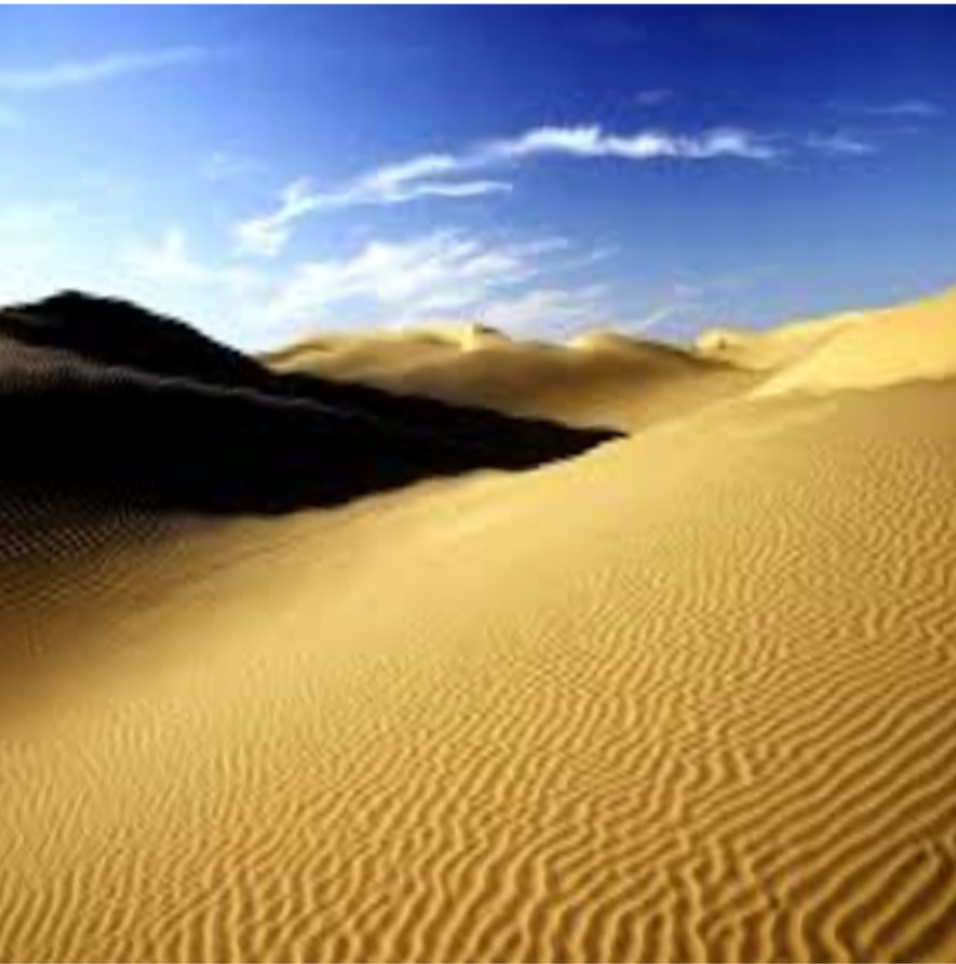#2017 iT 邦幫忙鐵人賽
DAY 9
1
Modern Web

## distortion

``````attribute vec2 a_textureCoords;
attribute vec4 a_position;

varying vec2 v_textureCoords;

attribute float a_pointSize;

void main() {
gl_Position = a_position;
gl_PointSize = 10.0;

v_textureCoords = a_textureCoords;
}
``````

#### 撰寫 distortion function

``````float distortion(float point,float freq, float speed) {
return sin(point * freq + ((3.1415/2.0) * u_time * speed));
}

``````

point 代表傳入的點位置，第二和第三個參數分別代表頻率與速度。我們這邊很簡單地回傳用 sin 處理過後的數值。因為我們要實現動畫效果，所以可以看到 `u_time` 這個變數，我們待會會讓 Javascript 傳值進來。

``````void main() {
vec2 distortions = distortions(v_textureCoords);
gl_FragColor = texture2D(u_sampler, vec2(distortions.x, distortions.y));
}
``````

``````precision mediump float;

varying vec2 v_textureCoords;

uniform float u_time;
uniform sampler2D u_sampler;
uniform vec2 u_resolution;

vec2 pixel() {
return 1.0 / u_resolution;
}

// make position distortion by sin func.
float distortion(float point,float freq, float speed) {
return sin(point * freq + ((3.1415/2.0) * u_time * speed));
}

vec2 distortions(vec2 pos) {
vec2 intensity = vec2(1.0,1.0) * pixel();

vec2 waves = vec2(
distortion(pos.y,100.0,0.35),
distortion(pos.x,100.0,0.4)
);

return pos + (waves * intensity * 1.0);
}

void main() {
vec2 distortions = distortions(v_textureCoords);

gl_FragColor = texture2D(u_sampler, vec2(distortions.x, distortions.y));
}
``````

### 撰寫 Javascript

``````const time = gl.getUniformLocation(program, 'u_time');
const resolution    = gl.getUniformLocation(program, 'u_resolution');
gl.uniform2f(resolution, canvas.width, canvas.height);
const textureCoords = gl.getAttribLocation(program, 'a_textureCoords');
const position      = gl.getAttribLocation(program, 'a_position');
const pointSize     = gl.getAttribLocation(program, 'a_pointSize');

const textures = [
0, 0,
1, 0,
0, 1,
1, 1,
];

const vertices = [
-0.8, -0.8,
0.8, -0.8,
-0.8, 0.8,
0.8, 0.8,
];
``````